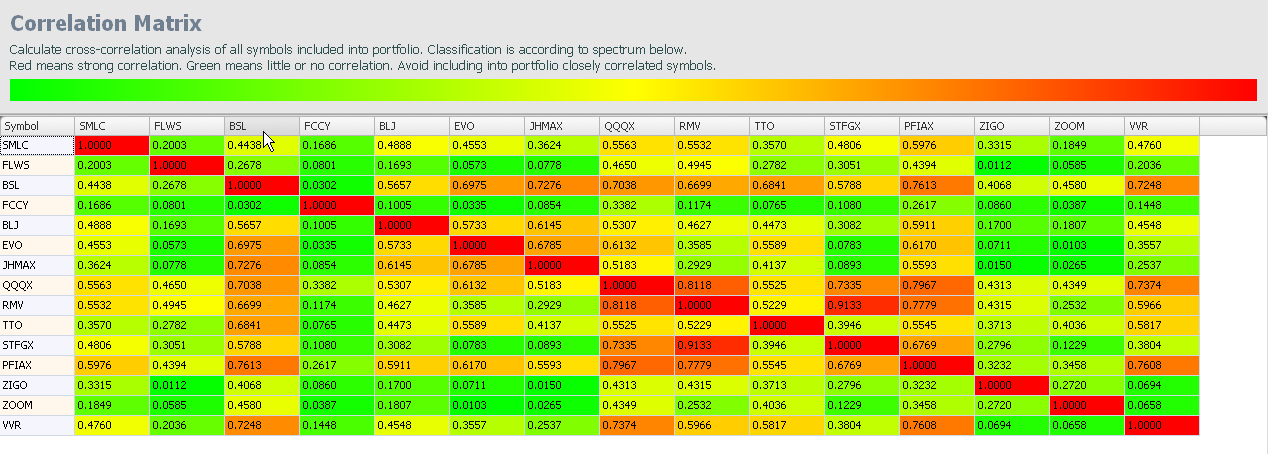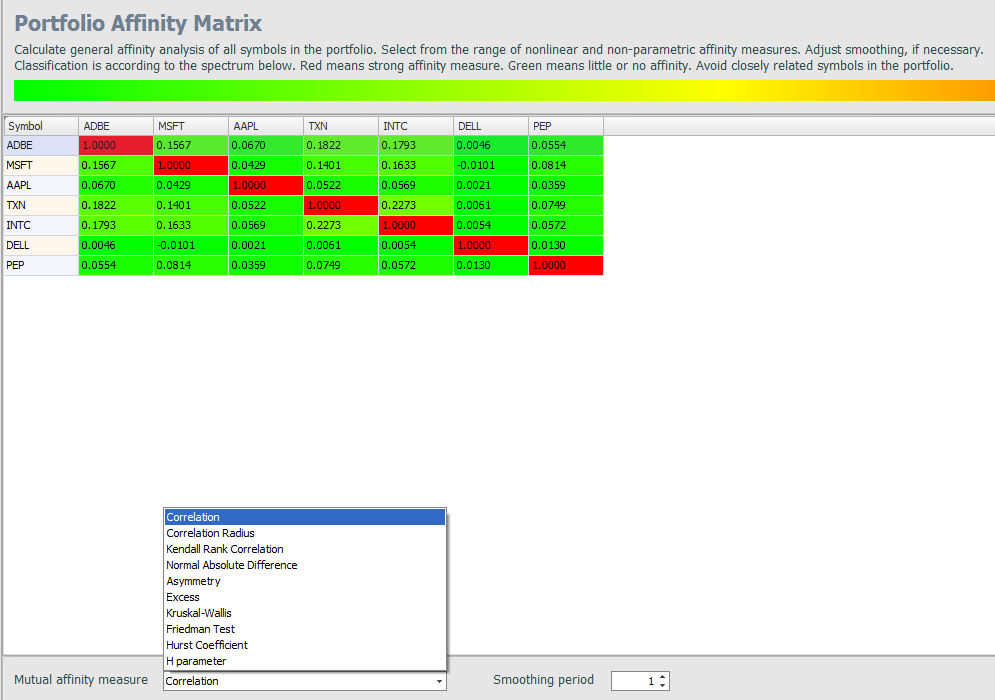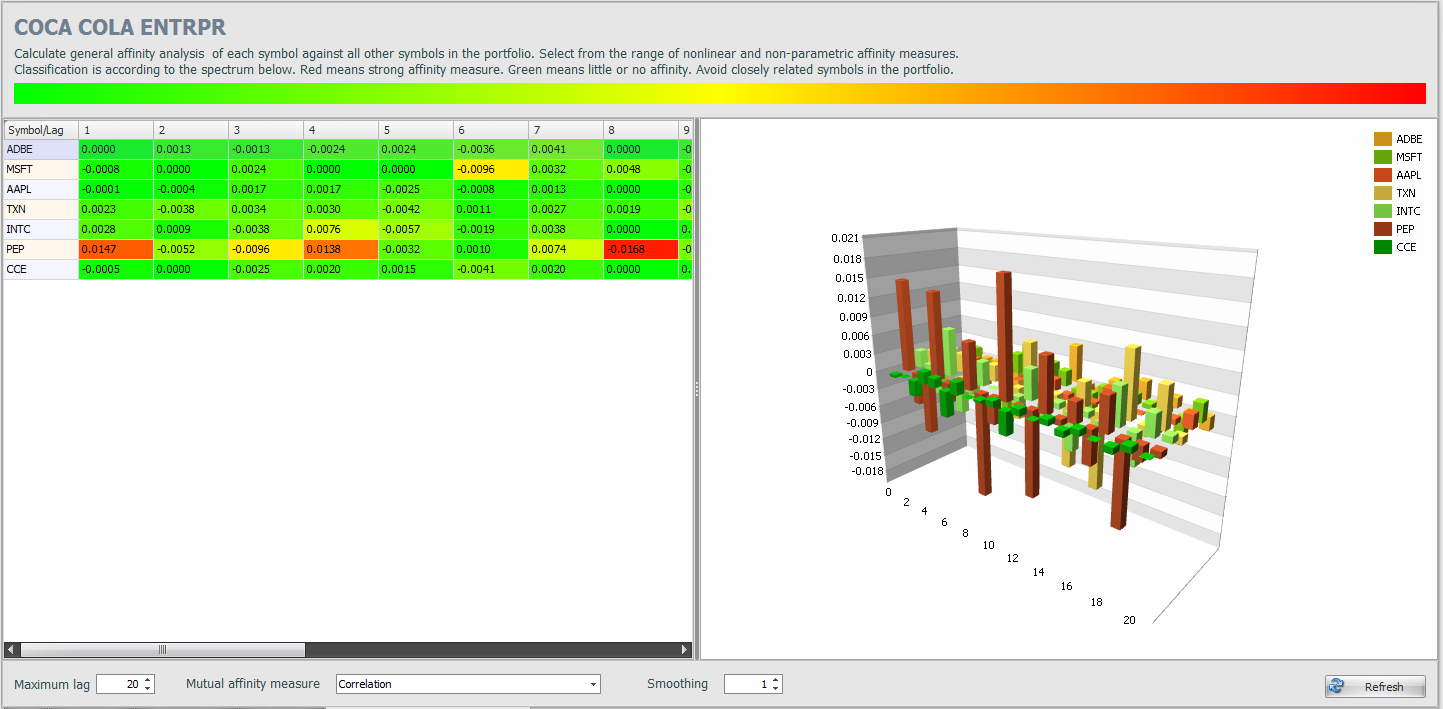# Portfolio optimization

Collective analysis of symbols for balancing of portfolio

Portfolio optimizer

• Summary view of all symbols in portfolio
• Forecasted price and change per symbol
• Distinctive coloring of signalsPortfolio affinity matrix

• Powerful tool for portfolio optimization
• Exclude closely correlated symbols
• Correlated symbols similarly behave on market changes
• It decreases stability of portfolio
• Keep portfolio of less correlated symbols
• Increase probability of some symbols growing while others downtrendMultiple affinity measures

• Kendall rank correlation
• Normal absolute difference
• Kruskal-Wallis test
• Friedman test
• Hurst coefficient
• H parameter
• Correlation
• Asymmetry
• ExcessLag relation analysis

• Discovery of symbol dependencies
• Calculates cross correlation of selected symbol with other symbols in portfolio over a range of time lags
• If strong correlation exists, then correlated symbol can serve as a predictor in multiple regression model
• Multiple classical and nonparametric proximity measures